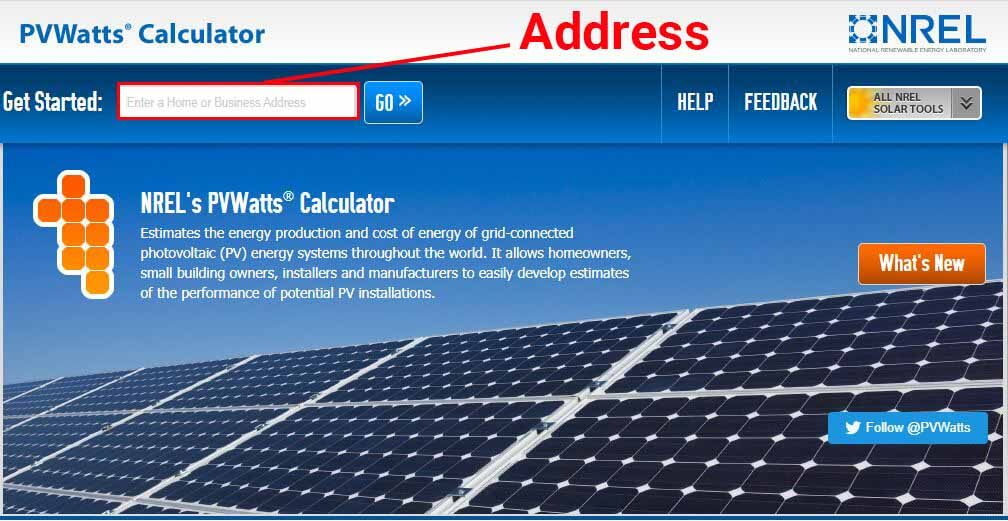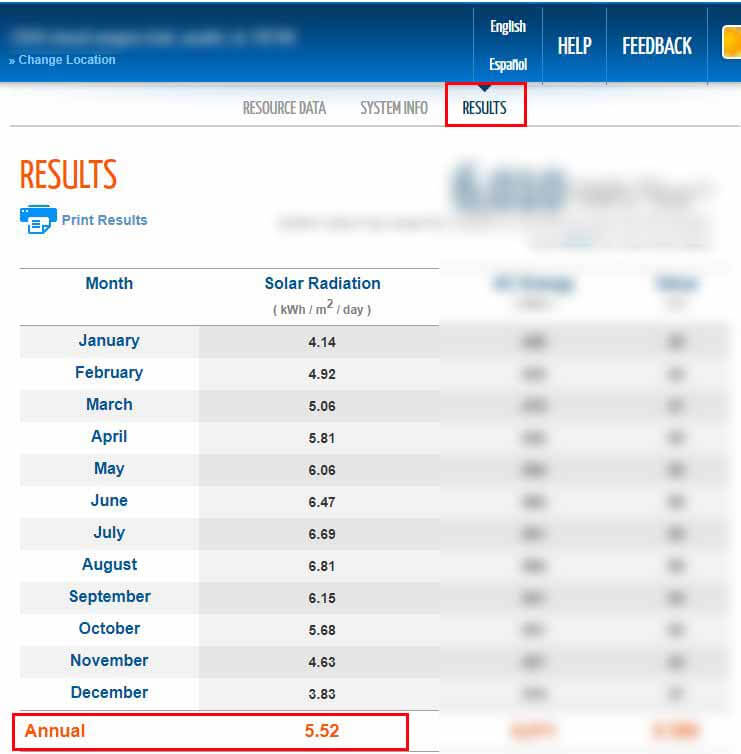# How many solar panels do I need for 1000 kwh per month?

A 1000 kWh per month is a reasonable amount of energy consumption, therefore, a solar system that could offset that will be of a reasonable size.

However, this isn’t a “one size fits all” situation. The size of the system and the number of solar panels necessary to produce this amount of energy, depend mainly on where the system is installed.

In this article, I’ll explain what this means, and I’ll show you how to perform your own estimations.

## How many solar panels do I need for 1,000 kWh per month?

Different residential solar panels have different outputs, ranging from 280 to 400 Watts each. A better way to ask this question is: how many kW of solar power do I need for 1000 kWh per month?

On average, you would need about 6.5 kW of solar power to produce 1000 kWh per month. In states such as Arizona, Nevada, or Kansas, where there’s an abundance of direct sunlight, a 5.5 kW system should be enough. However, in states such as Illinois, Massachusetts, and Connecticut, you might need as much as 7.5 kW.

The exact size of the system, and the number of solar panels required to produce 1000 kWh per month, differs from location to location.

This is because solar panels rely on direct sunlight to produce anything near their rated output. And other than weather conditions, the amount of direct sunlight that a solar panel receives mainly depends on where it is installed.

For example, a 5 kW solar installation in Austin, Texas, would – on average – produce 27 kWh of energy per day (820 kWh per month). In the summer, a system of this size and in this location could produce as much as 34 kWh per day.

Thanks to solar radiation data collected by geostationary satellites, we can predict the average amount of energy that a solar panel or an array of panels is capable of producing in a certain location.

If you know how much energy you need to produce (1000 kWh/month in this case), you can estimate the size of the system that can produce it. All you need is an estimate of the average amount of sunlight energy available to you. This amount of sunlight energy is referred to as Peak Sun Hours.

### What is Peak Sun Hours?

Peak Sun Hours represent the amount of sunlight energy that a location receives per unit of time. It can be used to estimate the amount of energy that a solar installation can potentially produce in a certain location.

Before I explain what this means, here are a couple of things to keep in mind:

• kW stands for kilo-Watts (1kW = 1000 Watts) and is the unit for measuring electrical power. For example, with enough sunlight, a 5 kW system is capable of producing 5 kW of power at a given moment.
• kWh stands for kilo-Watt-hours (1kWh = 1000 Wh) and is the unit of measuring electrical energy. In other words, this is the unit to measure how much energy is consumed or produced. For example, a solar panel that produces 100 Watts continuously for 5 hours, is said to have produced 500 Wh of energy (0.5 kWh).

The amount of sunlight that a certain area receives is measured in W/m² (Watts per Square Meter). For a solar panel to produce 100% of its rated power, it needs exactly 1000 W/m².

For example, a 5 kW solar installation can only produce 5 kW (5000 Watts) at a given moment if it receives 1000 W/m² of sunlight at that moment.

If it continues receiving  1000 W/m² of sunlight for 1 hour, it can be said to have received 1000 Wh/m² (1 kWh/m²) of sunlight energy, and it will have produced 5 kWh (5000 Watt-hours) of energy by the end of that hour.

In other words, our 5 kW installation can only produce 5 kWh of energy if it receives exactly 1 kWh/m² (1000 Wh/m²) of sunlight energy. 1 kWh/m² of sunlight energy is equivalent to 1 Peak Sun Hour.

If this installation received 3 Peak Sun Hours, it would have received 3 kWh/m² of energy from the sun, and would therefore have produced 15 kWh of energy.

So, logically, if you know the average Peak Sun Hours that you get per day, and how much energy you need to produce, you could calculate the size of the system that you need:

System Wattage (kW) = Daily Energy production (kWh) ÷ Daily Peak Sun Hours

System Wattage (kW) = Monthly Energy production (kWh) ÷ (Daily Peak Sun Hours x 30)

### How many Peak Sun Hours do you get?

You can use NREL’s PVWatts Calculator to determine the average daily Peak Sun Hour (kWh/m²/day) that you get in your location. All you need is an address.For example, I entered an address in Austin, TX, and the tool estimates that the annual average Peak Sun Hours in this location is 5.52. This means that on average, this location receives 5.52kWh/m² of sunlight energy per day.

Using the previous formula, the size of the system required to produce 1000 kWh/month is calculated as such:

System Wattage (kW) = Monthly Energy production (kWh) ÷ (Daily Peak Sun Hours x 30)

System Wattage (kW) = 1000 kWh ÷ (5.52 x 30)

System Wattage (kW) = 6.03 kW

The average residential solar panel is rated at 330 Watts (0.33 kW). So a system of this size (6.03 kW) would consist of about 18-20 solar panels.

To make things even easier, I have made a calculator that does these calculations for you.

## Solar panels kWh calculator

Based on your monthly energy consumption, and the annual average Peak Sun Hours that you get in your location, the following calculator will estimate the size of the system and the number of solar panels that you need.

Please note that in the winter, the average Peak Sun Hours that your location receives are lower than the yearly average. And it does make sense because some months and some days are sunnier than others.

However, using the annual average means that over 1 year, your system will on average generate 1000 kWh per month.

In some states, the excess energy generated in the summer can be sold to the utility company at full retail price and bought back in the winter at the same price. In these states, the grid can be used as a battery.

However, this is not the case for every state. Read up more on net metering policies here.

Another important thing to note is that in some cases, your roof might not have enough usable square footage to fit all the solar panels that you need. This can be due to roof orientation, surrounding trees, etc…

Read up more on this topic here: How many solar panels can I fit on my roof?